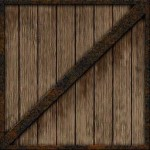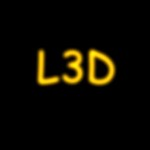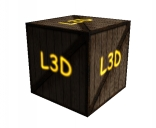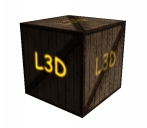Prev: Texture + Fragment Next: The Normal Matrix

Multitexturing is also really easy in GLSL. All we have to do is to access both textures. And since in here we’re going to use the same texture coordinates we don’t even have to rewrite the vertex shader. The fragment shader also suffers a minor change to add both textures colors.

```varying vec3 lightDir,normal;
uniform sampler2D tex,l3d;

void main()
{
vec3 ct,cf;
vec4 texel;
float intensity,at,af;
intensity = max(dot(lightDir,normalize(normal)),0.0);

cf = intensity * (gl_FrontMaterial.diffuse).rgb +
gl_FrontMaterial.ambient.rgb;
af = gl_FrontMaterial.diffuse.a;
texel = texture2D(tex,gl_TexCoord.st)+

texture2D(l3d,gl_TexCoord.st);
ct = texel.rgb;
at = texel.a;
gl_FragColor = vec4(ct * cf, at * af);

}```Texture Unit 0 Texture Unit 1 Textured Cube

And now for something a little different: a glow in the dark effect. We want the second texture to glow in the dark, i.e. it will be fully bright when the light doesn’t hit, and it will be dimmed as it gets more light.Additive Multi-Texture Glowing Multi-Texture

We have to recompute the final color in two steps: first we compute a color which is the first texture modulated with the fragments color, and afterwards we add the second texture unit depending on the intensity.

If intensity is zero then we want the second texture in its full strength. When the intensity is 1 we only want a 10% contribution of the second texture unit. For all the other values of intensity we want to interpolate. We can achieve this with the smoothstep function. This function has the following signature:

`	genType smoothStep(genType edge0, genType edge1, genType x);`

The result will be zero if x <= edge0, 1 if x >= edge1 and performs smooth Hermite interpolation between 0 and 1 when edge0 < x < edge1. In our case we want to call the function as follows:

`	coef = smoothStep(1.0, 0.2, intensity);`

The following fragment shader does the trick:

```varying vec3 lightDir,normal;
uniform sampler2D tex,l3d;

void main()
{
vec3 ct,cf,c;
vec4 texel;
float intensity,at,af,a;

intensity = max(dot(lightDir,normalize(normal)),0.0);

cf = intensity * (gl_FrontMaterial.diffuse).rgb +
gl_FrontMaterial.ambient.rgb;
af = gl_FrontMaterial.diffuse.a;

texel = texture2D(tex,gl_TexCoord.st);

ct = texel.rgb;
at = texel.a;

c = cf * ct;
a = af * at;

float coef = smoothstep(1.0,0.2,intensity);
c += coef *  vec3(texture2D(l3d,gl_TexCoord.st));

gl_FragColor = vec4(c, a);
}```

A Shader Designer project is available in here.

 Prev: Texture + Fragment Next: The Normal Matrix

### 3 Responses to “Multi-Texture”

1.Very concise work. Helps get started quickly. Thanks for all the efforts.

2.Great tutorial! Is it possible to have and example of how to have two textures, but each being shown at various positions or heights on the mesh?

3.Fantastic tutorials! They’ve really helped a lot. Thanks so much for the effort.

This site uses Akismet to reduce spam. Learn how your comment data is processed.